# Point By Point

We can draw the graph of a parabola by taking all the integer values ofshown on theaxis, calculate the correspondingvalue for each, plot each point in turn and join them with a smooth curve.This method is not very efficient.

# Using Special Points

Another way is to calculate the special points of the parabola and plot only those.

The special points are:

•-intercept
• vertex
•-intercepts (if there are any)
• symmetry of the-interceptThe special points can be calculated quite easily from the quadratic expression given in factored form or in completed square form.

# Special Points from Factored Form

## ExampleTheintercept occurs when thecoordinate is zero. Therefore when:The-intercept is the point.

The-intercepts occur when thecoordinate is zero. To locate them, we need to solve the equationWhen any two numbers multiply to give zero, one or the other or both of the numbers must be zero. Therefore, ifThe-intercepts occur at the pointsand.

Vertex: Thecoordinate of the vertex is right in the middle of the intercepts. To find the middle ofand, we can do a variety of calculations. One way is to add them together and divide by 2 (find the average of the two numbers).Now to find thecoordinate of the vertex, we simply substitute thecoordinate into the equation for. When:The coordinates of the vertex are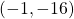.

Let’s plot the points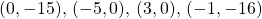:The last point to plot is the symmetry of theintercept. If you imagine a mirror line through the vertex, and reflect theintercept over that to plot a fifth point.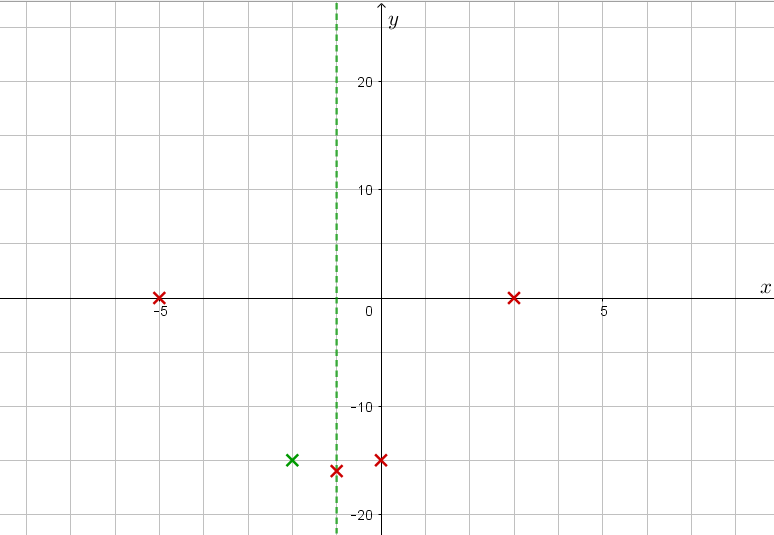We are now ready to draw a smooth curve through the plotted points, making sure to round off the vertex and not make it too pointy.# Special Points from Completed Square form:

We calculate the same special points, however our calculations look slightly different as our equation is presented differently.

Let’s graph the parabola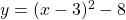.

The-intercept: The point on the parabola that lies on the-axis has. We substituteinto the equation:The-intercept has coordinatesThe-intercepts: We substituteand solve directly, remembering that when we take a square root we must remember to consider both the positive and the negative square root. For example, if we know thatwe know that bothandare solutions.Now we take the square root and consider both negative and positive: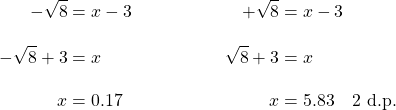Note that the exact value of these roots can be simplified:The-intercepts have coordinatesand.

The vertex: The lowest point on the parabola has the lowestvalue. The lowest square number is zero. Since, we require ‘something’ to be equal to zero.

In our case,. Solve. The vertex has thecoordinate.

To calculate thecoordinate, as for anycoordinate, we substitute thecoordinate into the equation:The coordinates of the vertex are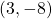.

Let’s plot the points,,andon the axes:…and draw the parabola:# Graph from standard formTo graph directly from the standard form, we need to employ processes that occur later in this unit. In summary:

To calculate theintercept, again we setand find that. Therefore theintercept has coordinates.

To calculate theintercepts, we need to use one of the following processes:

To calculate thecoordinate of the vertex, we need to use one of these processes:

• use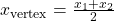once theintercepts are known;
• use the formula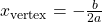;
• write the expression in completed square form

We then substitute thecoordinate into the equation to find thecoordinate of the vertex.

These processes are discussed in the rest of the unit.